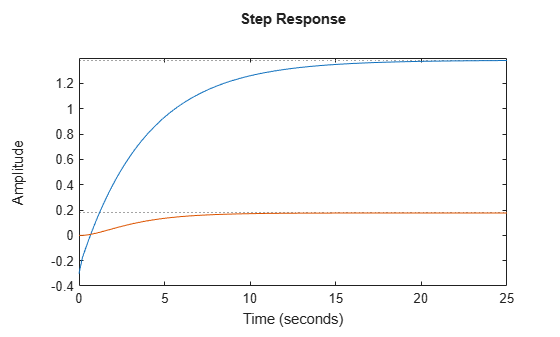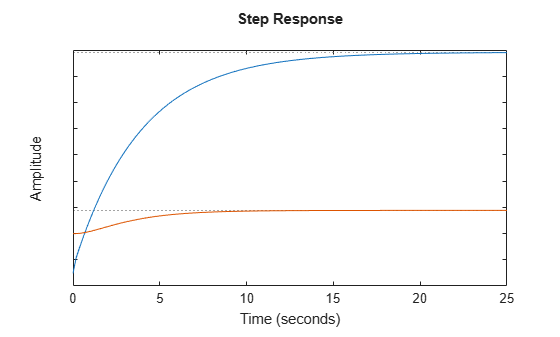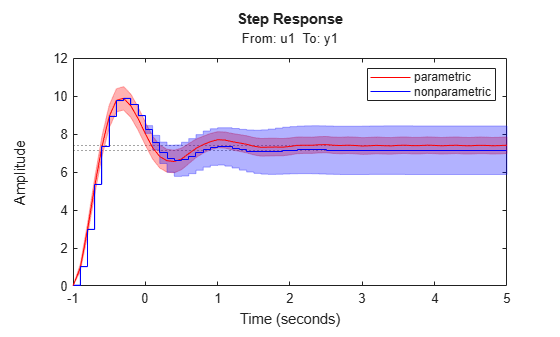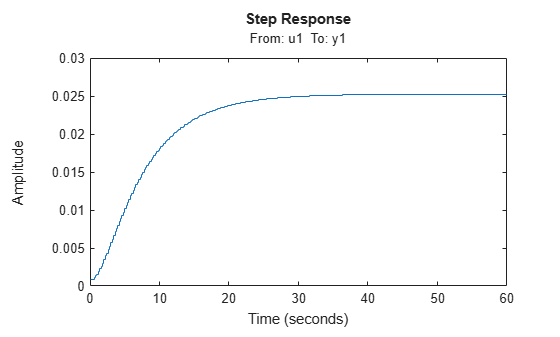Main Content

# stepplot

Plot step response and return plot handle

## Syntax

```h = stepplot(sys) stepplot(sys,Tfinal) stepplot(sys,t) stepplot(sys1,sys2,...,sysN) stepplot(sys1,sys2,...,sysN,Tfinal) stepplot(sys1,sys2,...,sysN,t) stepplot(AX,...) stepplot(..., plotoptions) stepplot(..., dataoptions) ```

## Description

`h = stepplot(sys)` plots the step response of the dynamic system model `sys`. It also returns the plot handle `h`. You can use this handle to customize the plot with the `getoptions` and `setoptions` commands. Type

```help timeoptions ```

for a list of available plot options.

For multiinput models, independent step commands are applied to each input channel. The time range and number of points are chosen automatically.

`stepplot(sys,Tfinal)` simulates the step response from `t = 0` to the final time ```t = Tfinal```. Express `Tfinal` in the system time units, specified in the `TimeUnit` property of `sys`. For discrete-time systems with unspecified sample time (`Ts = -1`), `stepplot` interprets `Tfinal` as the number of sampling intervals to simulate.

`stepplot(sys,t)` uses the user-supplied time vector `t` for simulation. Express `t` in the system time units, specified in the `TimeUnit` property of `sys`. For discrete-time models, `t` should be of the form `Ti:Ts:Tf`, where `Ts` is the sample time. For continuous-time models, `t` should be of the form `Ti:dt:Tf`, where `dt` becomes the sample time of a discrete approximation to the continuous system (see `step`). The `stepplot` command always applies the step input at `t=0`, regardless of `Ti`.

To plot the step responses of multiple models `sys1`,`sys2`,... on a single plot, use:

`stepplot(sys1,sys2,...,sysN)`

`stepplot(sys1,sys2,...,sysN,Tfinal)`

`stepplot(sys1,sys2,...,sysN,t)`

You can also specify a color, line style, and marker for each system, as in

```stepplot(sys1,'r',sys2,'y--',sys3,'gx') ```

`stepplot(AX,...)` plots into the axes with handle `AX`.

`stepplot(..., plotoptions)` customizes the plot appearance using the options set, `plotoptions`. Use `timeoptions` to create the options set.

`stepplot(..., dataoptions)` specifies options such as the step amplitude and input offset using the options set, `dataoptions`. Use `stepDataOptions` to create the options set.

## Examples

collapse all

Generate a step response plot for two dynamic systems.

```sys1 = rss(3); sys2 = rss(3); h = stepplot(sys1,sys2);```Each step response settles at a different steady-state value. Use the plot handle to normalize the plotted response.

`setoptions(h,'Normalize','on')`Now, the responses settle at the same value expressed in arbitrary units.

Compare the step response of a parametric identified model to a nonparametric (empirical) model, and view their 3-σ confidence regions. (Identified models require System Identification Toolbox™ software.)

Identify a parametric and a nonparametric model from sample data.

```load iddata1 z1 sys1 = ssest(z1,4); sys2 = impulseest(z1);```

Plot the step responses of both identified models. Use the plot handle to display the 3-σ confidence regions.

```t = -1:0.1:5; h = stepplot(sys1,sys2,t); showConfidence(h,3) legend('parametric','nonparametric')```The nonparametric model `sys2` shows higher uncertainty.

Load data for estimating a nonlinear Hammerstein-Wiener model.

```load(fullfile(matlabroot,'toolbox','ident','iddemos','data','twotankdata')); z = iddata(y,u,0.2,'Name','Two tank system');```

`z` is an `iddata` object that stores the input-output estimation data.

Estimate a Hammerstein-Wiener Model of order [1 5 3] using the estimation data. Specify the input nonlinearity as piecewise linear and output nonlinearity as one-dimensional polynomial.

`sys = nlhw(z,[1 5 3],pwlinear,poly1d);`

Create an option set to specify input offset and step amplitude level.

`opt = stepDataOptions('InputOffset',2,'StepAmplitude',0.5);`

Plot the step response until 60 seconds using the specified options.

`stepplot(sys,60,opt);`## Tips

You can change the properties of your plot, for example the units. For information on the ways to change properties of your plots, see Ways to Customize Plots.

## See Also

Introduced before R2006a

## Support

#### Learn how to automatically tune PID controller gains

Download code examples